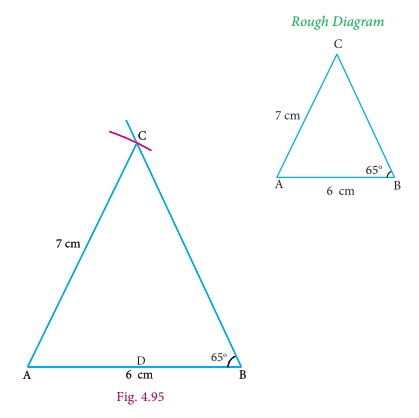Home | | Maths 9th std | Construction of the Incircle of a Triangle

# Construction of the Incircle of a Triangle

The incentre is (one of the triangle’s points of concurrency formed by) the intersection of the triangle’s three angle bisectors.

Construction of the Incircle of a Triangle

### Incentre

The incentre is (one of the triangle’s points of concurrency formed by) the intersection of the triangle’s three angle bisectors.The incentre is the centre of the incircle ; It is usually denoted by I; it is the one point in the triangle whose distances to the sides are equal.

### Example 4.15

Construct the incentre of ΔABC with AB = 6 cm, B = 65° and AC = 7 cm Also draw the incircle and measure its radius.

Solution

Step 1 : Draw the ΔABC with AB = 6cm, B = 65° and AC = 7cmStep 2 : Construct the angle bisectors of any two angles (A and B) and let them meet at I.

Then I  is the incentre of ΔABC. Draw perpendicular from I to any one of the side (AB) to meet AB at D.

Step 3: With I as centre and ID as radius draw the circle. This circle touches all the sides of the triangle internally.